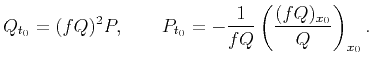Time-to-depth conversion and seismic velocity estimation using time-migration velocityNext: 3-D case Up: Partial differential equations for Previous: Partial differential equations for

## 2-D case

Consider a set of image rays coming to the surface. Suppose we are tracing them all backwards in time together with the quantitiesand. Let us eliminate the unknown velocityin system 9 using equation 10. Moreover, let us eliminate the differentiation inusing the definition ofand rewrite it in the time-domain coordinates. Indeed,, hence. Therefore, system 9 becomes(13)

Eliminatingin system 13, we get the following partial differential equation (PDE) for(14)

The initial conditions are,. Equation 14 simplifies in terms of the negative reciprocal of. Introduce. Then equation 14 becomes(15)

In the expanded form, equation 15 is(16)Time-to-depth conversion and seismic velocity estimation using time-migration velocityNext: 3-D case Up: Partial differential equations for Previous: Partial differential equations for

2013-03-02